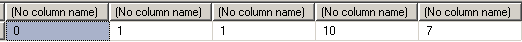SQL Server and T-SQL Development Tutorials Development resources, articles, tutorials, code samples and tools and downloads for ASP.Net, SQL Server, R Script, Windows, Windows Phone, AWS, SAP HANA and ABAP, like SAP UI5, Screen Personas, etc.
 Home Articles News IT Jobs Tools Sample Chapters Trainers Blogs Forums Photos Files

Install SQL Server 2019

# Fuzzy String Matching using Levenshtein Distance Algorithm in SQL Server

The Levenshtein distance algoritm is a popular method of fuzzy string matching.
Levenshtein distance algorithm has implemantations in SQL Server also.
Levenshtein distance sql functions can be used to compare strings in SQL Server by t-sql developers.

The term Levenshtein distance between two strings means the number of character replacements or chararacter insert or character deletion required to transform one string to other.

Levenshtein distance is also known as Edit Distance.

If two strings are equal the Levenstein distance is 0, zero.
A zero value for Levenshtein distance between two string variables in SQL Server means, these two string variables are identical.

The higher the value of Levenstein distance between two varchar or nvarchar string variables means the strings are more different than each other. As the Levenstein distance algoritm counts each character edition to transform one string to other, if strings are completely different then the Levenstein distance function will result high values.

The return of a SQL Levenstein distance function is an integer.

The name Levenshtein is for the memory of Vladimir Levenshtein who is the developer of this idea.

One of the most used SQL Levenshtein distance among sql programmers is as follows:
Please note that the code is taken from a forum post at SQLTeam.

``` CREATE FUNCTION edit_distance(@s1 nvarchar(3999), @s2 nvarchar(3999)) RETURNS int AS BEGIN  DECLARE @s1_len int, @s2_len int  DECLARE @i int, @j int, @s1_char nchar, @c int, @c_temp int  DECLARE @cv0 varbinary(8000), @cv1 varbinary(8000)  SELECT   @s1_len = LEN(@s1),   @s2_len = LEN(@s2),   @cv1 = 0x0000,   @j = 1, @i = 1, @c = 0  WHILE @j <= @s2_len   SELECT @cv1 = @cv1 + CAST(@j AS binary(2)), @j = @j + 1  WHILE @i <= @s1_len  BEGIN   SELECT    @s1_char = SUBSTRING(@s1, @i, 1),    @c = @i,    @cv0 = CAST(@i AS binary(2)),    @j = 1   WHILE @j <= @s2_len   BEGIN    SET @c = @c + 1    SET @c_temp = CAST(SUBSTRING(@cv1, @j+@j-1, 2) AS int) +     CASE WHEN @s1_char = SUBSTRING(@s2, @j, 1) THEN 0 ELSE 1 END    IF @c > @c_temp SET @c = @c_temp    SET @c_temp = CAST(SUBSTRING(@cv1, @j+@j+1, 2) AS int)+1    IF @c > @c_temp SET @c = @c_temp    SELECT @cv0 = @cv0 + CAST(@c AS binary(2)), @j = @j + 1  END  SELECT @cv1 = @cv0, @i = @i + 1  END  RETURN @c END ```

Please note that this sql function is developed by Joseph Gama.

Here is the outputs of sample Levenshtein distance sql function for SQL Server developers.

``` select  dbo.edit_distance('Fuzzy String Match','fuzzy string match'),  dbo.edit_distance('fuzzy','fuzy'),  dbo.edit_distance('Fuzzy String Match','fuzy string match'),  dbo.edit_distance('levenshtein distance sql','levenshtein sql server'),  dbo.edit_distance('distance','server') ```Related SQL Resources

SQL Server Articles

SQL Server 2012

SQL Server Tools

SQL Blog

MS SQL Server Forums